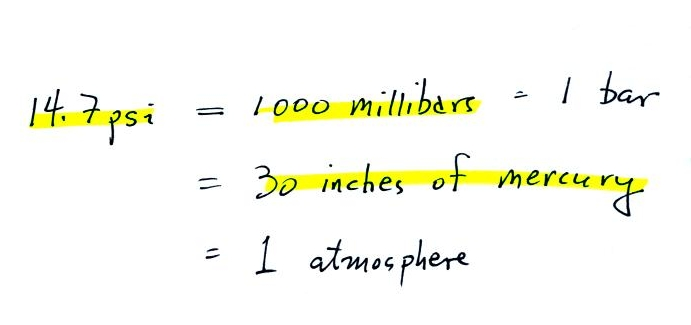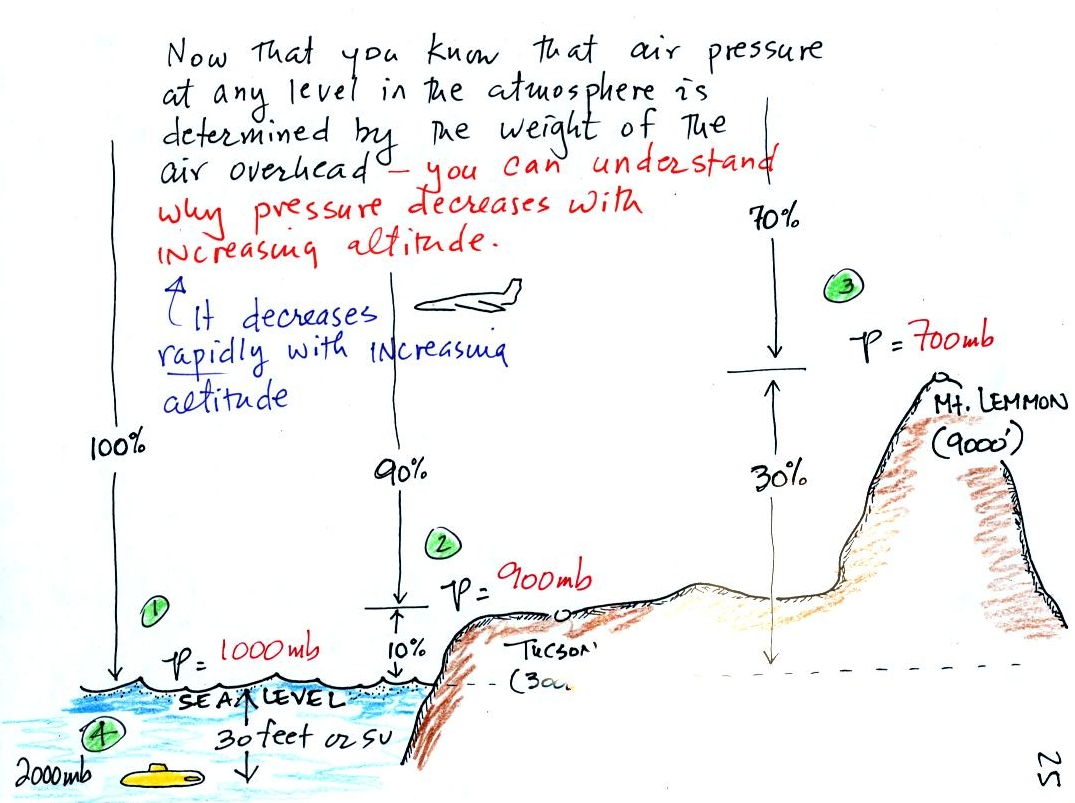Pressure is defined as force divided by area.  Air pressure is the weight of the atmosphere overhead divided by the area the air is resting on.  Atmospheric pressure is determined by and tells you something about the weight of the air overhead.  This is one way, a sort of large scale representation, of understanding air pressure.  At some point later in the semester you'll learn about the ideal gas law which is a 2nd, microscopic, way of understanding air pressure.  (the figures below differ somewhat from those drawn in class but emphasize the same points)Under normal conditions a 1 inch by 1 inch column of air stretching from sea level to the top of the atmosphere will weigh 14.7 pounds.  Normal atmospheric pressure at sea level is 14.7 pounds per square inch (psi, the units you use when you fill up your car or bike tires with air).A steel bar was passed around in the classroom.  The steel bar also weighs exactly 14.7 pounds (many people think it is heavier than that).  Steel is a lot denser than air, so a steel bar only needs to be 52 inches tall to have the same weight as an air column that is 100 miles or more tall.  If you were to stand the bar on end it would push against the ground with a pressure of 14.7 psi.

Here are some of the other commonly used pressure units.Typical sea level pressure is 14.7 psi or about 1000 millibars (the units used by meterologists and the units that you will use in this class most of the time) or about 30 inches of mercury (refers to the reading on a mercury barometer).

Pressure at sea level is determined by the weight of the air overhead.  What happens to pressure as you move upward in the atmosphere.  We can use a pile of bricks to help answer this question.  I use bricks because you can see them.  You can think of the bricks representing layers of air in the atmosphere.Each brick weighs 5 pounds.  At the bottom of the 5 brick tall pile you would measure a weight of 25 pounds.  If you moved up a brick you would measure a weight of 20 pounds, the weight of the four bricks still above.  To get the pressure you would need to divide by the area.  It should be clear that weight and pressure will decrease as you move up the pile.

In the atmosphere, pressure at any level is determined by the weight of the air still overhead.  Pressure decreases with increasing altitude because there is less and less air remaining overhead.  The figure is a more carefully drawn version of what was done in class.At sea level altitude, at Point 1, the pressure is normally about 1000 mb.  That is determined by the weight of all (100%) of the air in the atmosphere.

Some parts of Tucson, at Point 2, are 3000 feet above sea level (most of the valley is a little lower than that around 2500 feet).  At 3000 ft. about 10% of the air is below, 90% is still overhead.  It is the weight of the 90% that is still above that determines the atmospheric pressure in Tucson.  If 100% of the atmosphere produces a pressure of 1000 mb, then 90% will produce a pressure of 900 mb.

Pressure is typically about 700 mb at the summit of Mt. Lemmon (9000 ft. altitude at Point 3) and 70% of the atmosphere is overhead..

Pressure decreases rapidly with increasing altitude.  We will find that pressure changes more slowly if you move horizontally.  But the small horizontal changes are what cause the wind to blow and what cause storms to form.

Point 4 wasn't mentioned in class but shows a submarine at a depth of about 30 ft.  The pressure there is determined by the weight of the air and the weight of the water overhead.  Water is much denser and much heavier than air.  At 33 ft., the pressure is already twice what it would be at the surface of the ocean (2000 mb instead of 1000 mb).